Wekinator

# How to Play Music with an Arduino Accelerometer and Gyroscope Sensor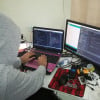November 09, 2018 by Muhammad Aqib
Share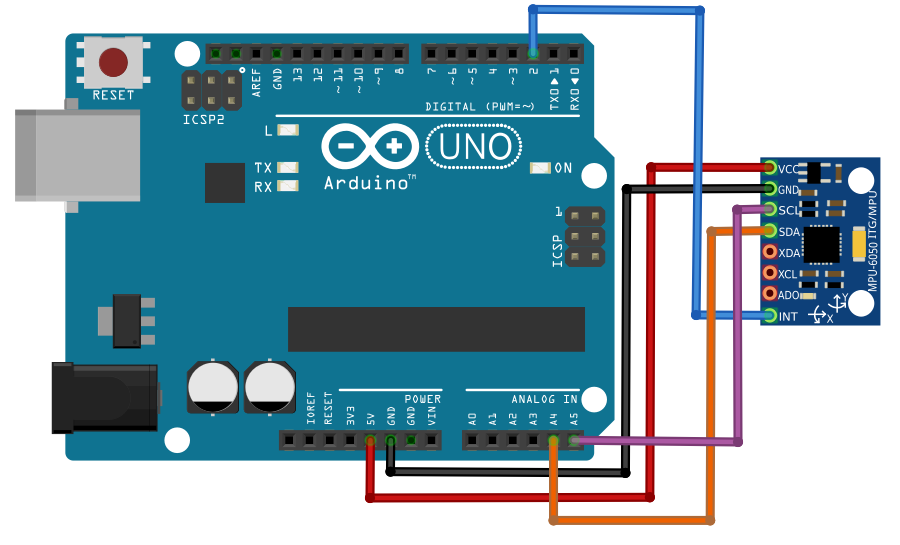Learn how to play music using an accelerometer and gyroscope sensor with the Wekinator software platform.

In this article, you'll learn how to generate music through an accelerometer and an MPU6050 gyroscope (also referred to as a "gyro sensor"), using the Wekinator open-source software platform to enable machine learning techniques.

For a helpful introduction to the Wekinator platform, check out our Machine Learning for Makers: How to Get Started With Wekinator article.

The first step of this project is to interface the MPU6050 sensor with an Arduino, which will send the output data to processing.

In processing, we'll calculate the YPR (yaw, pitch, roll) values and draw a 3D model that mimics the movement of the sensor then sends the values to the Wekinator. Finally, the output data is sent to a drum machine made in processing that produces music.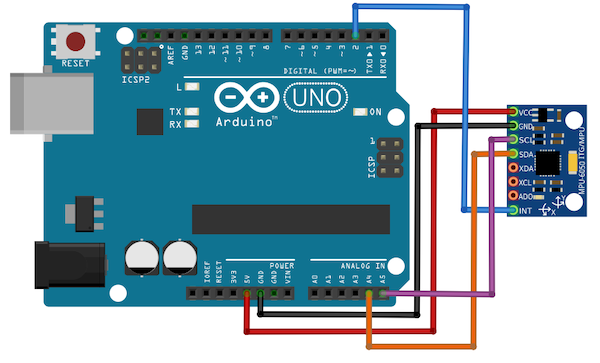Directions to connect the MPU6050 gyroscope to an Arduino.

### The Input

On the input side, the sensor will need to be connected to the Arduino. The directions for doing so are shown in the figure below.

### Installing the Arduino Libraries

To properly interface the Arduino with the sensor, first download the Arduino library developed for that purpose. You'll also require the I2C library, which you can access through this file folder

Next, unzip the contents of the file, open the folder titled, "Arduino" and move the I2C and MPU6050 sensor folder to the Arduino library folder.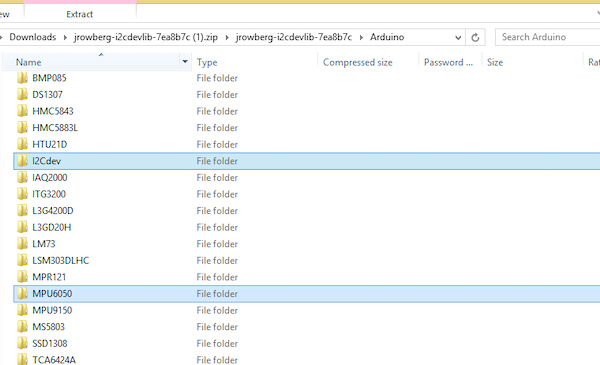Example indicating the selected locations of the I2C dev and MPU6050 folders.

Start by launching the Arduino IDE software. Navigate to examples under 'File', then MPU6050 and open the MPU6050_DMP6 file.Example demonstrating how to navigate to MPU6050_DMP6 file in Arduino IDE.

Upload the code found in the Arduino IDE and it should display on the serial monitor.

If it's showing the output data, then you can assume the sensor has successfully interfaced with the Arduino.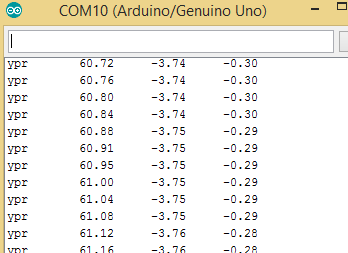Output data displayed in a window confirming the MPU605 sensor has successfully connected interfaced with the Arduino.

To send the data to processing, some changes need to be made to the code.

First, select the line 117 of the code and uncomment it, move up to line 100 and comment that segment of code. If you have trouble with this step, reference the figure below.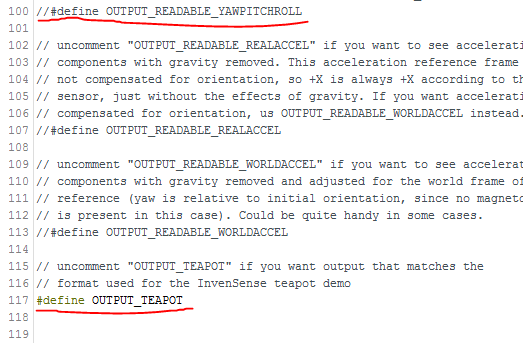An example underlining the lines of code that need to be changed.

If you upload the code again, it will display unreadable characters on the serial monitor.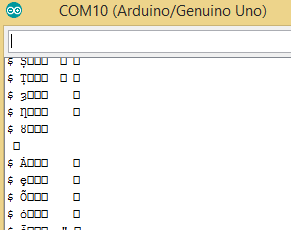An example of unreadable character code on the serial monitor window.

#### Processing Code

In order to receive the data from the Arduino, you'll be using the 'toxiclibs' libraryReference image indicating the circled location of the 'toxiclibs' library file.

Next, copy the entire folder into the zip file and paste it in the processing library folder. You'll navigate to 'yourProcessingFolder', modes, java, then the libraries category.

Now paste the code provided below (a modified version of the example in the sensor's library) into processing and upload it.

``````import processing.serial.*;
import processing.opengl.*;
import toxi.geom.*;
import toxi.processing.*;
import oscP5.*;
import netP5.*;

OscP5 oscP5;

ToxiclibsSupport gfx;

Serial port;                         // The serial port
char[] teapotPacket = new char;  // InvenSense Teapot packet
int serialCount = 0;                 // current packet byte position
int synced = 0;
int interval = 0;

float[] q = new float;
Quaternion quat = new Quaternion(1, 0, 0, 0);

float[] gravity = new float;
float[] euler = new float;
float[] ypr = new float;

void setup() {
// 300px square viewport using OpenGL rendering
size(300, 300, OPENGL);
gfx = new ToxiclibsSupport(this);

// setup lights and antialiasing
lights();
smooth();

// display serial port list for debugging/clarity
println(Serial.list());

// get the first available port (use EITHER this OR the specific port code below)
String portName = Serial.list();

// get a specific serial port (use EITHER this OR the first-available code above)
//String portName = "COM4";

// open the serial port
port = new Serial(this, portName, 115200);

// send single character to trigger DMP init/start
// (expected by MPU6050_DMP6 example Arduino sketch)
port.write('r');

/* start oscP5, sending messages at port 9000 */
oscP5 = new OscP5(this,9000);
}

void draw() {
if (millis() - interval > 1000) {
// resend single character to trigger DMP init/start
// in case the MPU is halted/reset while applet is running
port.write('r');
interval = millis();
}

// black background
background(0);

// translate everything to the middle of the viewport
pushMatrix();
translate(width / 2, height / 2);

// 3-step rotation from yaw/pitch/roll angles (gimbal lock!)
// ...and other weirdness I haven't figured out yet
//rotateY(-ypr);
//rotateZ(-ypr);
//rotateX(-ypr);

// toxiclibs direct angle/axis rotation from quaternion (NO gimbal lock!)
// (axis order [1, 3, 2] and inversion [-1, +1, +1] is a consequence of
// different coordinate system orientation assumptions between Processing
// and InvenSense DMP)
float[] axis = quat.toAxisAngle();
rotate(axis, -axis, axis, axis);

// draw main body in red
fill(255, 0, 0, 200);
box(10, 10, 200);

// draw front-facing tip in blue
fill(0, 0, 255, 200);
pushMatrix();
translate(0, 0, -120);
rotateX(PI/2);
drawCylinder(0, 20, 20, 8);
popMatrix();

// draw wings and tail fin in green
fill(0, 255, 0, 200);
beginShape(TRIANGLES);
vertex(-100,  2, 30); vertex(0,  2, -80); vertex(100,  2, 30);  // wing top layer
vertex(-100, -2, 30); vertex(0, -2, -80); vertex(100, -2, 30);  // wing bottom layer
vertex(-2, 0, 98); vertex(-2, -30, 98); vertex(-2, 0, 70);  // tail left layer
vertex( 2, 0, 98); vertex( 2, -30, 98); vertex( 2, 0, 70);  // tail right layer
endShape();
vertex(-100, 2, 30); vertex(-100, -2, 30); vertex(  0, -2, -80); vertex(  0, 2, -80);
vertex( 100, 2, 30); vertex( 100, -2, 30); vertex(  0, -2, -80); vertex(  0, 2, -80);
vertex(-100, 2, 30); vertex(-100, -2, 30); vertex(100, -2,  30); vertex(100, 2,  30);
vertex(-2,   0, 98); vertex(2,   0, 98); vertex(2, -30, 98); vertex(-2, -30, 98);
vertex(-2,   0, 98); vertex(2,   0, 98); vertex(2,   0, 70); vertex(-2,   0, 70);
vertex(-2, -30, 98); vertex(2, -30, 98); vertex(2,   0, 70); vertex(-2,   0, 70);
endShape();

popMatrix();

//Send the OSC message
sendOsc();
}

void serialEvent(Serial port) {
interval = millis();
while (port.available() > 0) {

if (synced == 0 && ch != '\$') return;   // initial synchronization - also used to resync/realign if needed
synced = 1;
print ((char)ch);

if ((serialCount == 1 && ch != 2)
|| (serialCount == 12 && ch != '\r')
|| (serialCount == 13 && ch != '\n'))  {
serialCount = 0;
synced = 0;
return;
}

if (serialCount > 0 || ch == '\$') {
teapotPacket[serialCount++] = (char)ch;
if (serialCount == 14) {
serialCount = 0; // restart packet byte position

// get quaternion from data packet
q = ((teapotPacket << 8) | teapotPacket) / 16384.0f;
q = ((teapotPacket << 8) | teapotPacket) / 16384.0f;
q = ((teapotPacket << 8) | teapotPacket) / 16384.0f;
q = ((teapotPacket << 8) | teapotPacket) / 16384.0f;
for (int i = 0; i < 4; i++) if (q[i] >= 2) q[i] = -4 + q[i];

// set our toxilibs quaternion to new data
quat.set(q, q, q, q);

// below calculations unnecessary for orientation only using toxilibs

// calculate gravity vector
gravity = 2 * (q*q - q*q);
gravity = 2 * (q*q + q*q);
gravity = q*q - q*q - q*q + q*q;

// calculate Euler angles
euler = atan2(2*q*q - 2*q*q, 2*q*q + 2*q*q - 1);
euler = -asin(2*q*q + 2*q*q);
euler = atan2(2*q*q - 2*q*q, 2*q*q + 2*q*q - 1);

// calculate yaw/pitch/roll angles
ypr = atan2(2*q*q - 2*q*q, 2*q*q + 2*q*q - 1);
ypr = atan(gravity / sqrt(gravity*gravity + gravity*gravity));
ypr = atan(gravity / sqrt(gravity*gravity + gravity*gravity));

// output various components for debugging
//println("q:\t" + round(q*100.0f)/100.0f + "\t" + round(q*100.0f)/100.0f + "\t" + round(q*100.0f)/100.0f + "\t" + round(q*100.0f)/100.0f);
//println("euler:\t" + euler*180.0f/PI + "\t" + euler*180.0f/PI + "\t" + euler*180.0f/PI);
println("ypr:\t" + ypr*180.0f/PI + "\t" + ypr*180.0f/PI + "\t" + ypr*180.0f/PI);

}
}
}
}

float angle = 0;
float angleIncrement = TWO_PI / sides;
for (int i = 0; i < sides + 1; ++i) {
angle += angleIncrement;
}
endShape();

// If it is not a cone, draw the circular top cap
angle = 0;
beginShape(TRIANGLE_FAN);

// Center point
vertex(0, 0, 0);
for (int i = 0; i < sides + 1; i++) {
angle += angleIncrement;
}
endShape();
}

// If it is not a cone, draw the circular bottom cap
angle = 0;
beginShape(TRIANGLE_FAN);

// Center point
vertex(0, tall, 0);
for (int i = 0; i < sides + 1; i++) {
angle += angleIncrement;
}
endShape();
}
}

void sendOsc() {
OscMessage msg = new OscMessage("/wek/inputs");
oscP5.send(msg, dest);
}``````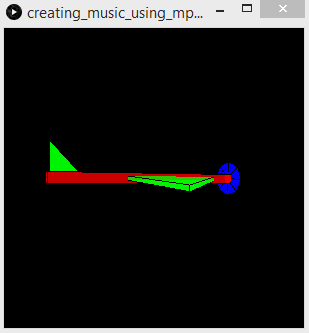### The Output

In terms of the output for this project, we'll be using a processing sketch that receives the output from the Wekinator and produces music according to its instructions.

The specific sketch will receive three, continuous, outputs from the Wekinator and is found on the examples page on the Wekinator website.

Download the 'Simple continuously-controlled drum machine' file from the webpage and run the sketch in the processing window.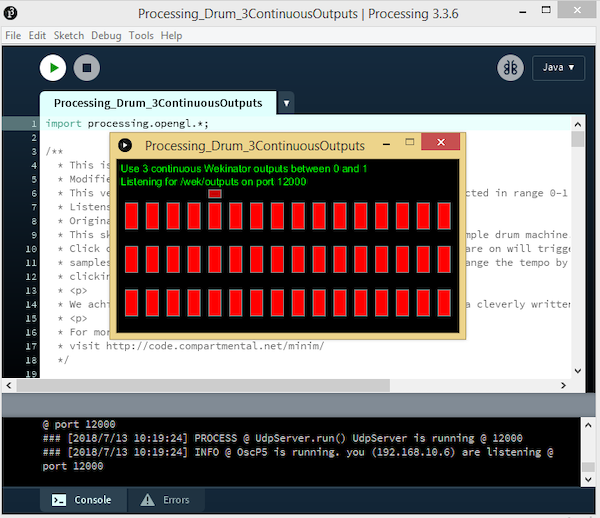Example of running the processing sketch in processing.

### Using Wekinator

In the Wekinator software, you'll need to set the settings to reflect those shown in the example below.

Assign the inputs values to 2 and outputs to 3. Also, designate the output type to the default setting, 'All continuous.'

This will prompt the Wekinator to send the 3 different outputs required to processing and cause different music to play, depending on the values.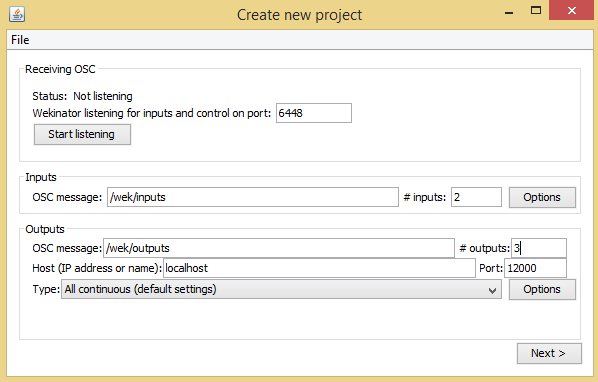If you click on the next button, you'll come to the 'New Project" window shown below.Set the output1 value to '1' and try tilting the sensor in any direction. Then, set the other two output boxes to 'o' and start the recording in order to create some audio samples.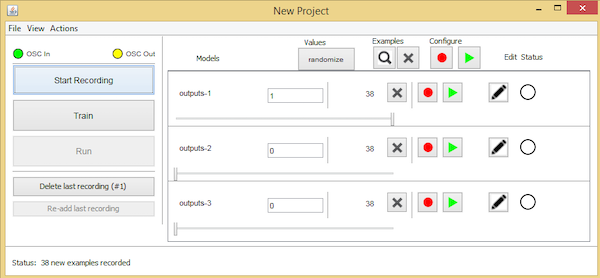Tilt the sensor in the other direction and set output2 to '1' and the other two outputs areas to '0', before starting to record a sample again.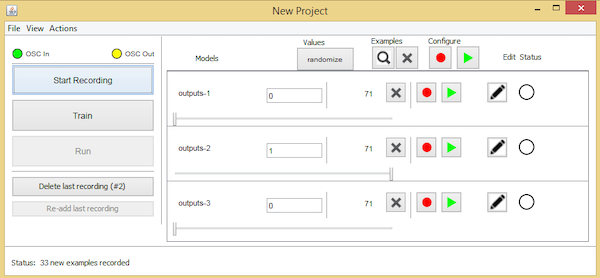Finally, tilt the sensor in another direction, of your choice and set output3 to '1' and the other two to '0'.

Record a final sample to examine the result of those particular value settings.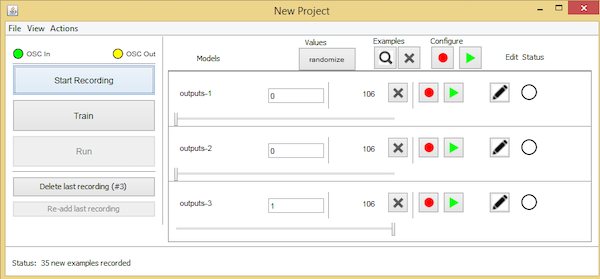Feel free to experiment further by recording additional samples while tilting the sensor in different directions.

After recording the sample, program the Wekinator and run it. It should produce music according to the sensor's movement.